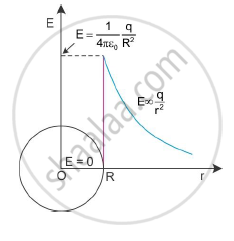Share

# Find the electric field intensity due to a uniformly charged spherical shell at a point, inside the shell. Plot the graph of electric field with distance from the centre of the shell. - CBSE (Science) Class 12 - Physics

ConceptUniformly Charged Infinite Plane Sheet and Uniformly Charged Thin Spherical Shell (Field Inside and Outside)

#### Question

Find the electric field intensity due to a uniformly charged spherical shell at a point (ii) inside the shell. Plot the graph of electric field with distance from the centre of the shell.

#### Solution

Field inside the shell: The point P will be inside the shell. The Gaussian surface is again a sphere through P centred at O.

The flux through the Gaussian surface, calculated as before, is E × 4πr2.

However, in this case, the Gaussian surface encloses no charge. Gauss’s law then gives

E = 4πr2 = 0

∴ E = 0

Thus, the field due to a uniformly charged thin shell is zero at all points inside the shell

Graph showing the electric field with the distance from the centre of the shell:Is there an error in this question or solution?

#### Video TutorialsVIEW ALL 

Solution Find the electric field intensity due to a uniformly charged spherical shell at a point, inside the shell. Plot the graph of electric field with distance from the centre of the shell. Concept: Uniformly Charged Infinite Plane Sheet and Uniformly Charged Thin Spherical Shell (Field Inside and Outside).
S